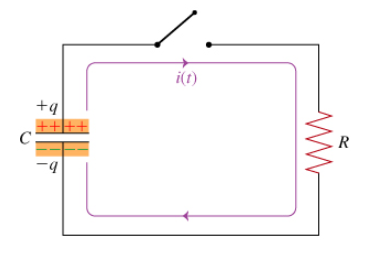# Problem: A capacitor with capacitance C is initially charged with charge q. At time t = 0, a switch is thrown to close the circuit connecting the capacitor in series with a resistor of resistance R. (Intro 1 figure)What is the current I0 that flows through the resistor immediately after theswitch is thrown?

###### FREE Expert Solution

We'll use Ohm's law to determine the current flowing through the resistor immediately after the switch is thrown.

Ohm's law:

$\overline{){\mathbit{i}}{\mathbf{=}}\frac{\mathbf{V}}{\mathbf{R}}}$###### Problem Details

A capacitor with capacitance C is initially charged with charge q. At time t = 0, a switch is thrown to close the circuit connecting the capacitor in series with a resistor of resistance R. (Intro 1 figure)What is the current I0 that flows through the resistor immediately after theswitch is thrown?